Topics: Back to homepage, wavelet transform, discrete wavelet transform, continuous wavelet transform, wavelet denoising, wavelet compression

## Wavelet denoising

Discrete wavelet transform can be used for easy and fast denoising of a noisy signal. If we take only a limited number of highest coefficients of the discrete wavelet transform spectrum, and we perform an inverse transform (with the same wavelet basis) we can obtain more or less denoised signal. There are several ways how to choose the coefficients that will be keeped. Here, only two most simple methods were tried - hard and soft tresholding. In the Fig. 1 one can see a simple signal (sine with a lineary increasing frequency) that was used for most of the demonstrations. In the next examples, a noise was added to this signal and the denoising procedure ws used. For this denoising, approximately 4 % of the wavelet coefficients were used for the reconstruction of the original signal.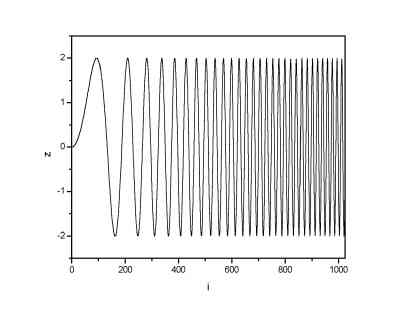Original signal

Example 1

The uniform noise was added to the 1024 points long signal.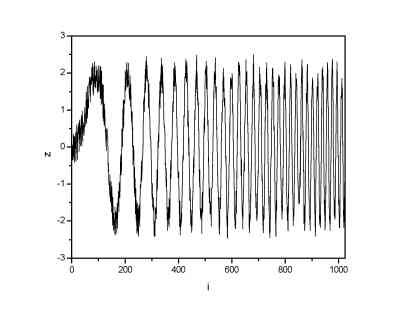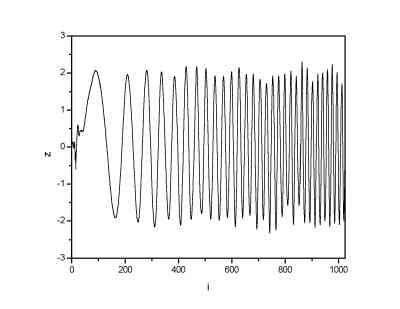Signal with uniform noise (noise range (-0.5; 0.5)) Signal denoised by means of DWT

Example 2

The uniform noise was added to the 65536 points long signal.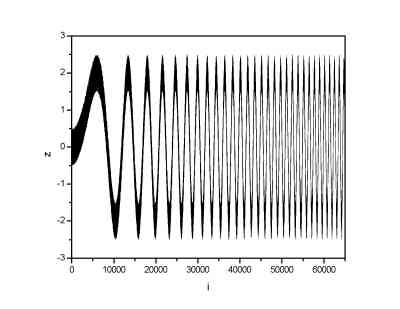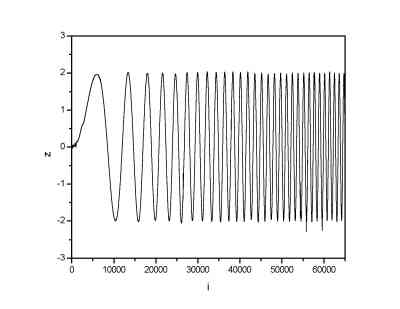Signal with uniform noise (noise range (-0.5; 0.5)) Signal denoised by means of DWT

Example 3

Gaussian noise with sigma=0.2 was added to the 1024 points long signal.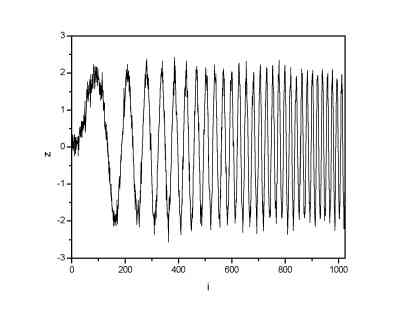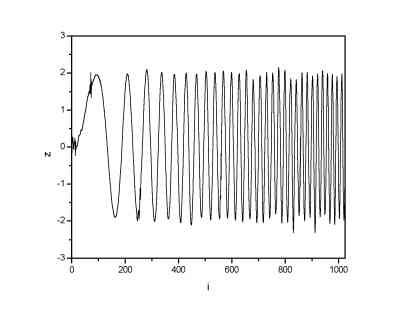Signal with gaussian noise (sigma=0.2) Signal denoised by means of DWT

Example 4

Gaussian noise with sigma=0.5 was added to the 1024 points long signal.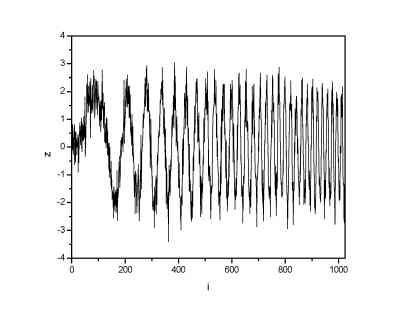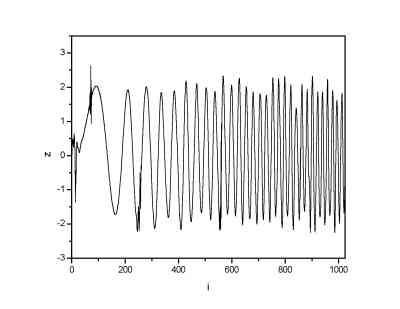Signal with gaussian noise (sigma=0.5) Signal denoised by means of DWT

Example 5

Gaussian noise with sigma=1 was added to the 1024 points long signal.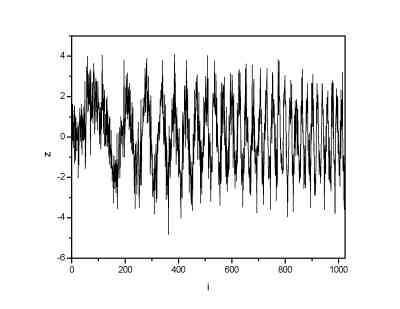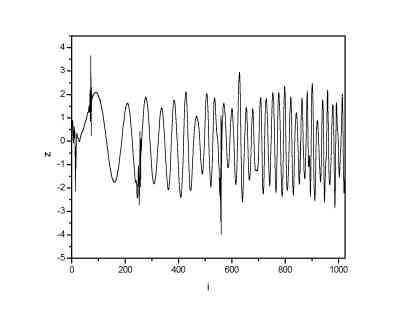Signal with gaussian noise (sigma=1) Signal denoised by means of DWT

Wavelet type influence

As it is known from the theory of discrete wavelet transform, the choice of the proper wavelet scaling function is allways the most important thing. Generally, for the denoising the wavelet scaling function should have properties similar to the original signal (continuity, continuity in derivatives etc.). Here, the effects of using two different wavelets were compared. We used the Daubechies 8 and Daubechies 20 wavelet. Note that for both the wavelets the denoising procedure resulted in quite satisfying result. If we use for example Haar wavelet (box scaling function) for our signal constructed from sine functions, the result would be very poor. As a signal we used a sine function which was in some parts of the signal multiplied by a constant.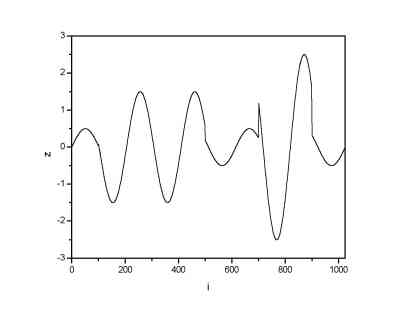Original signal

We than added a gaussian noise to this function by a similar way as in previous examples. Example 6

Here denoised signals are plotted for two basis wavelets. The original signal is plotted as well (red line).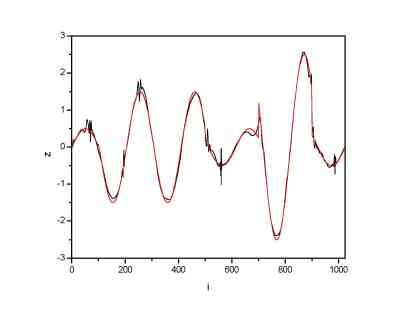Denoising using Daubechies 8 scaling function Denoising using Daubechies 20 scaling function
We can see that in both cases signal was reconstructed in a satisfactory way. The comparison between the difference between the signals and the original one is given below.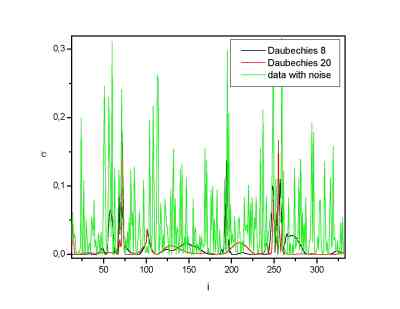Square deviation of the noisy and denoised signals with a respect to the original one: the yellow lines give the noisy results, e.g. the noise. The other two lines are the denoised signals. Only part of whole signal is plotted to see the differences better. the big suppression of the noise in the denoised data is seen. The Daubechies 20 wavelet scaling function gives better results here. Also the standard deviation of the denoised signal with a respect to the original one is for this (Daubechies 20) wavelet approximately 30 % lower than for the Daubechies 8 wavelet.

Real data examples

Created by Petr Klapetek, February 2002